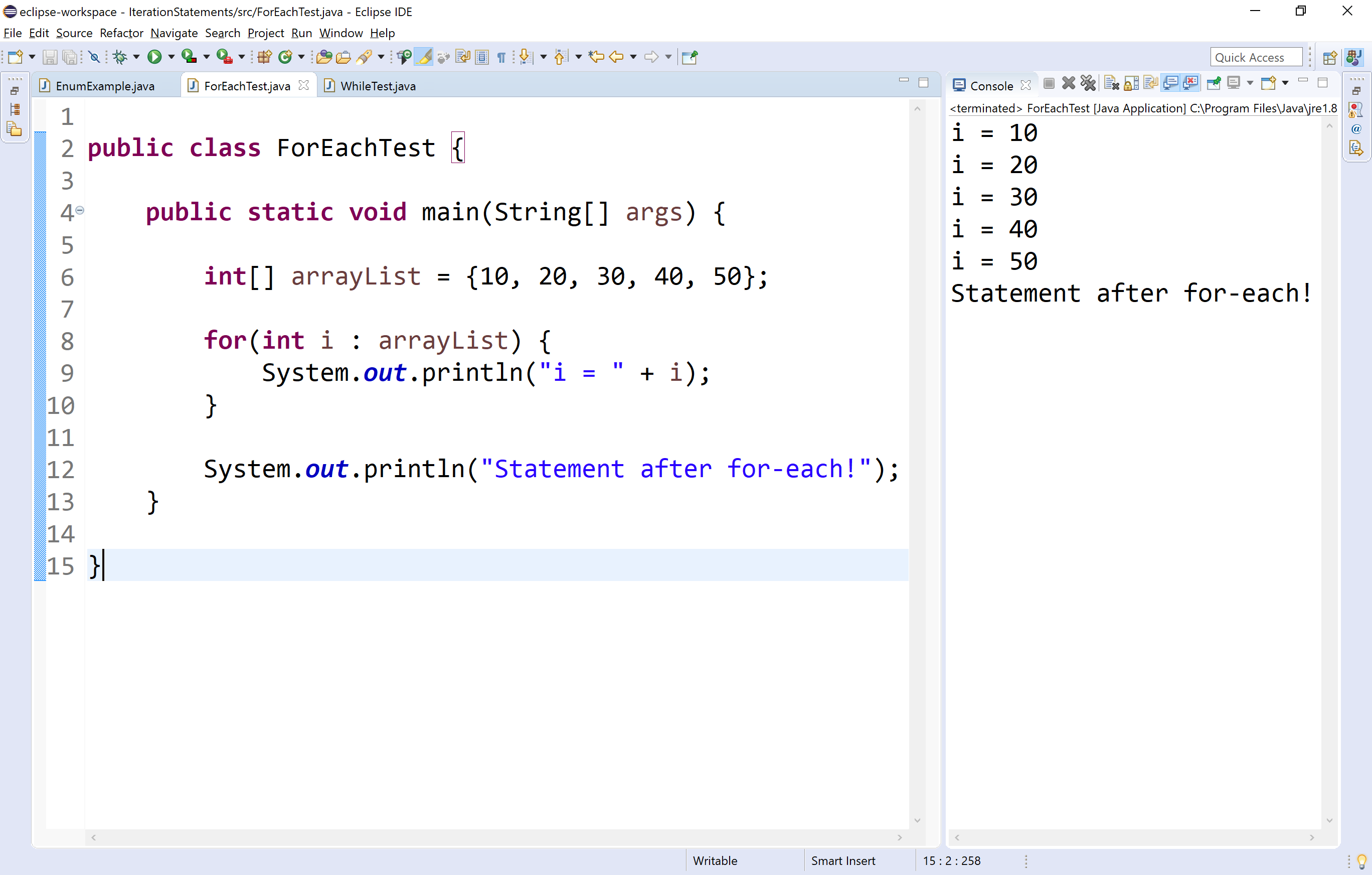The perfect place for easy learning...

×

# Java Iterative Statements

The java programming language provides a set of iterative statements that are used to execute a statement or a block of statements repeatedly as long as the given condition is true. The iterative statements are also known as looping statements or repetitive statements. Java provides the following iterative statements.

• while statement
• do-while statement
• for statement
• for-each statement

## while statement in java

The while statement is used to execute a single statement or block of statements repeatedly as long as the given condition is TRUE. The while statement is also known as Entry control looping statement. The syntax and execution flow of while statement is as follows.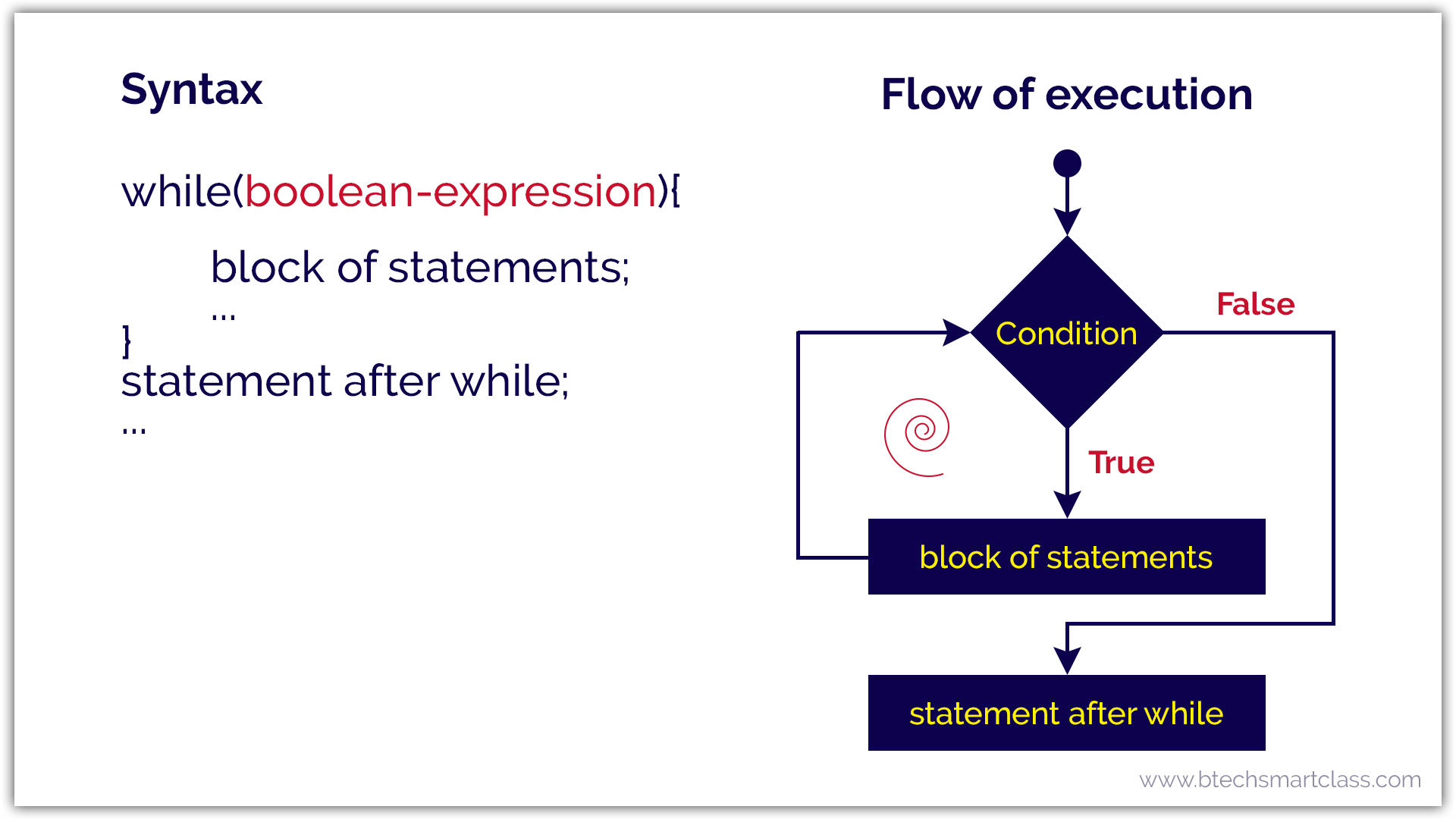Let's look at the following example java code.

Java Program
``````ipublic class WhileTest {

public static void main(String[] args) {

int num = 1;

while(num <= 10) {
System.out.println(num);
num++;
}

System.out.println("Statement after while!");

}

}```
```

When we run this code, it produce the following output.## do-while statement in java

The do-while statement is used to execute a single statement or block of statements repeatedly as long as given the condition is TRUE. The do-while statement is also known as the Exit control looping statement. The do-while statement has the following syntax.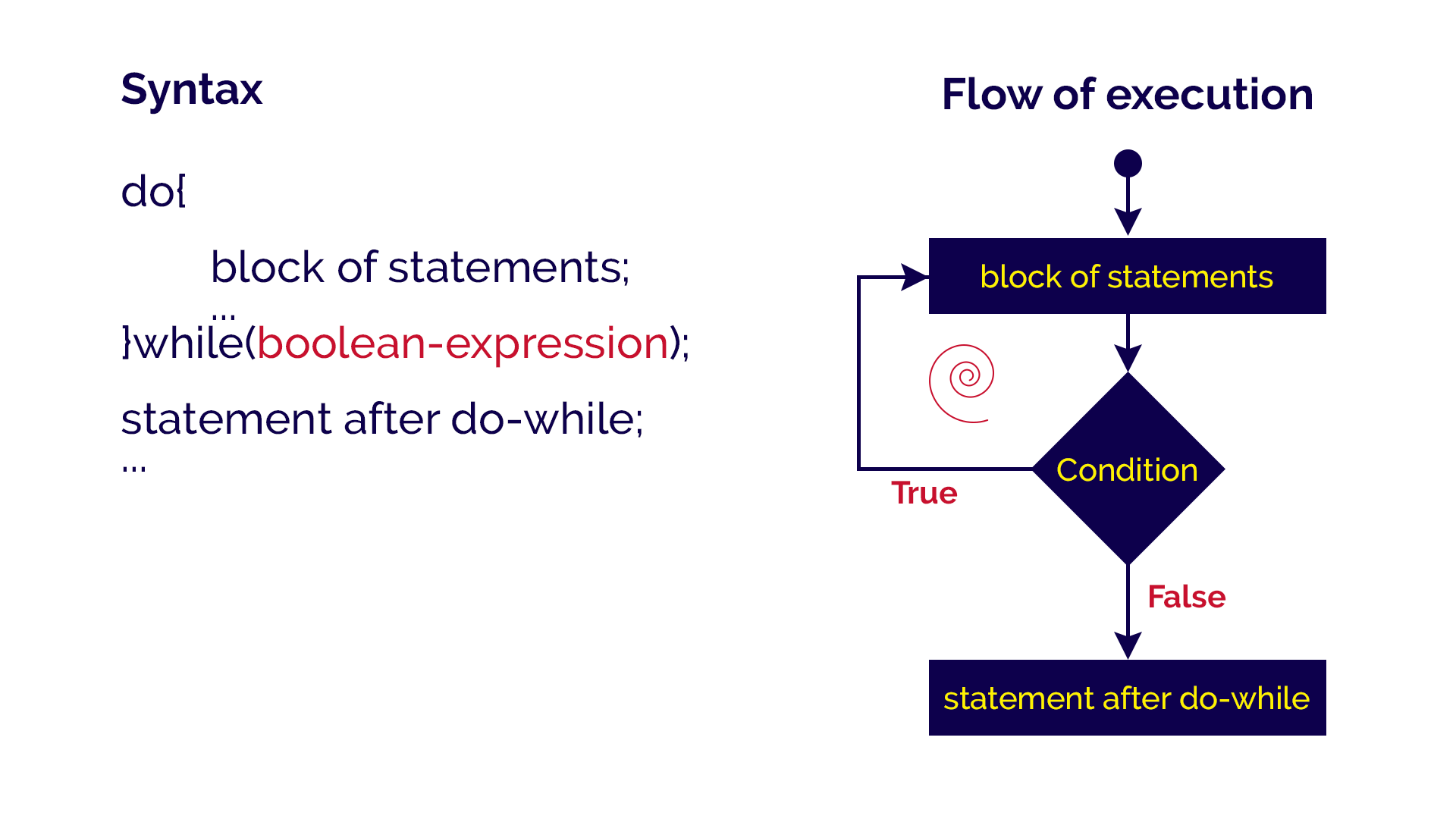Let's look at the following example java code.

Java Program
``````public class DoWhileTest {

public static void main(String[] args) {

int num = 1;

do {
System.out.println(num);
num++;
}while(num <= 10);

System.out.println("Statement after do-while!");

}

}```
```

When we run this code, it produce the following output.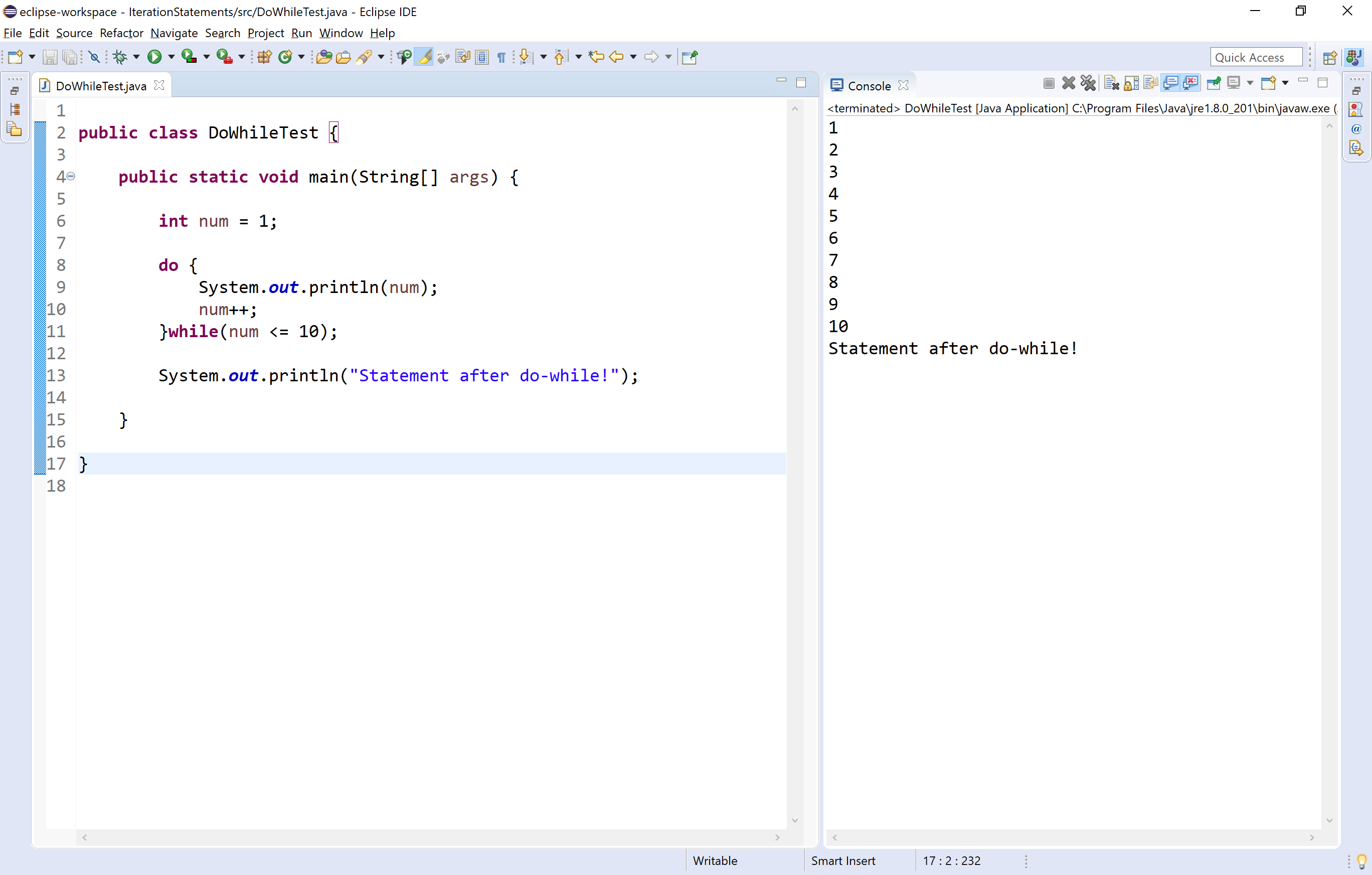## for statement in java

The for statement is used to execute a single statement or a block of statements repeatedly as long as the given condition is TRUE. The for statement has the following syntax and execution flow diagram.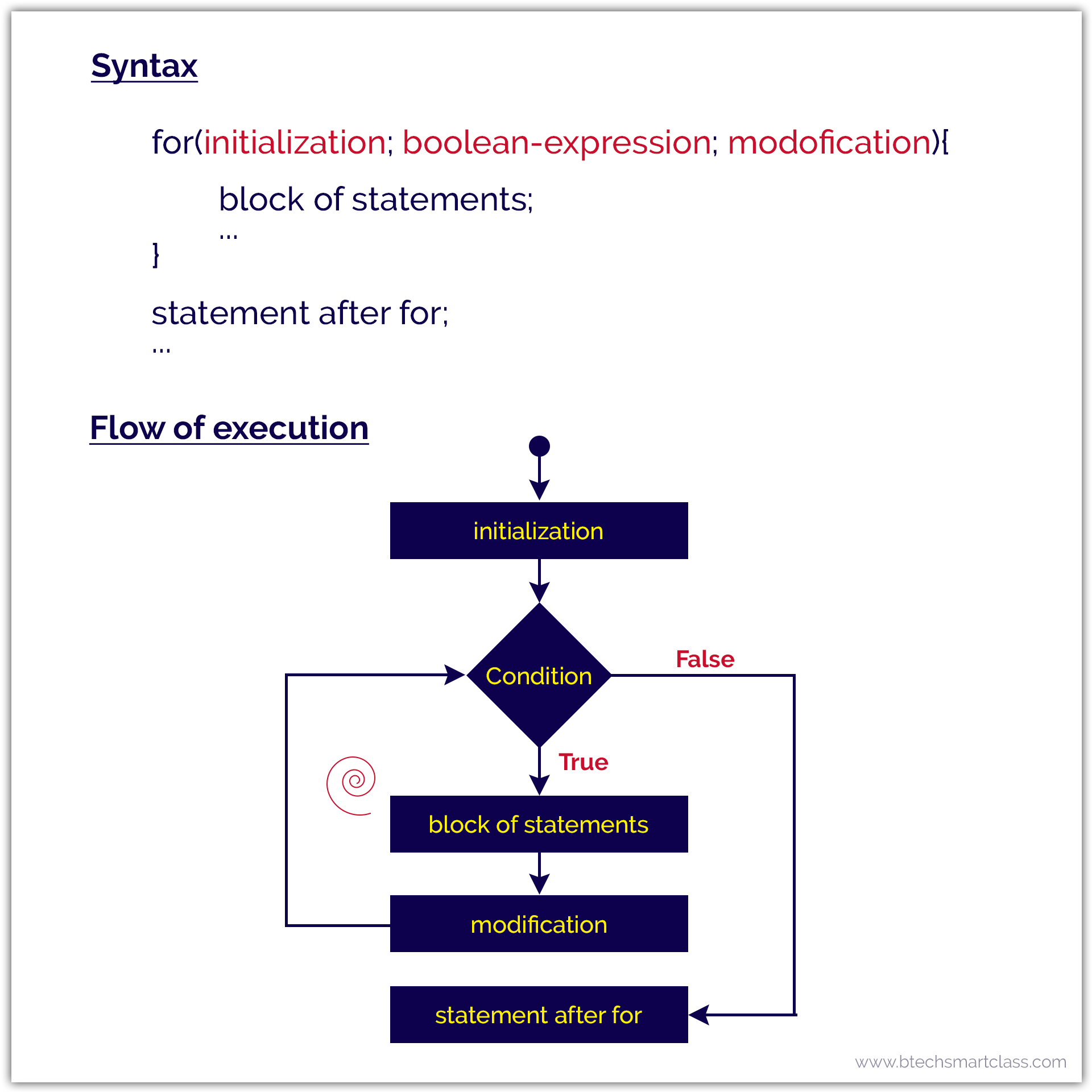In for-statement, the execution begins with the initialization statement. After the initialization statement, it executes Condition. If the condition is evaluated to true, then the block of statements executed otherwise it terminates the for-statement. After the block of statements execution, the modification statement gets executed, followed by condition again.

Let's look at the following example java code.

Java Program
``````public class ForTest {

public static void main(String[] args) {

for(int i = 0; i < 10; i++) {
System.out.println("i = " + i);
}

System.out.println("Statement after for!");
}

}```
```

When we run this code, it produce the following output.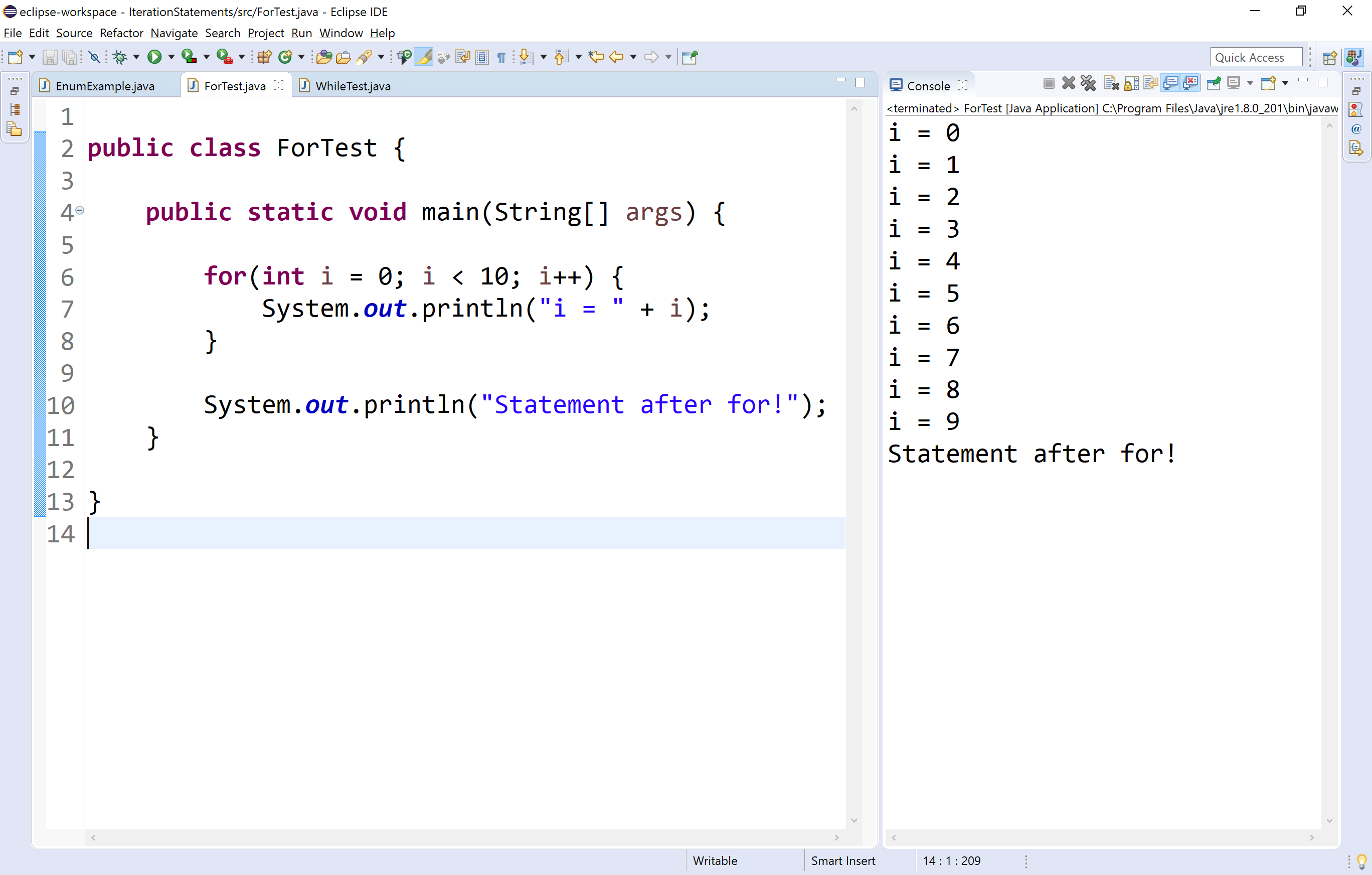## for-each statement in java

The Java for-each statement was introduced since Java 5.0 version. It provides an approach to traverse through an array or collection in Java. The for-each statement also known as enhanced for statement. The for-each statement executes the block of statements for each element of the given array or collection.

🔔 In for-each statement, we can not skip any element of given array or collection.

The for-each statement has the following syntax and execution flow diagram.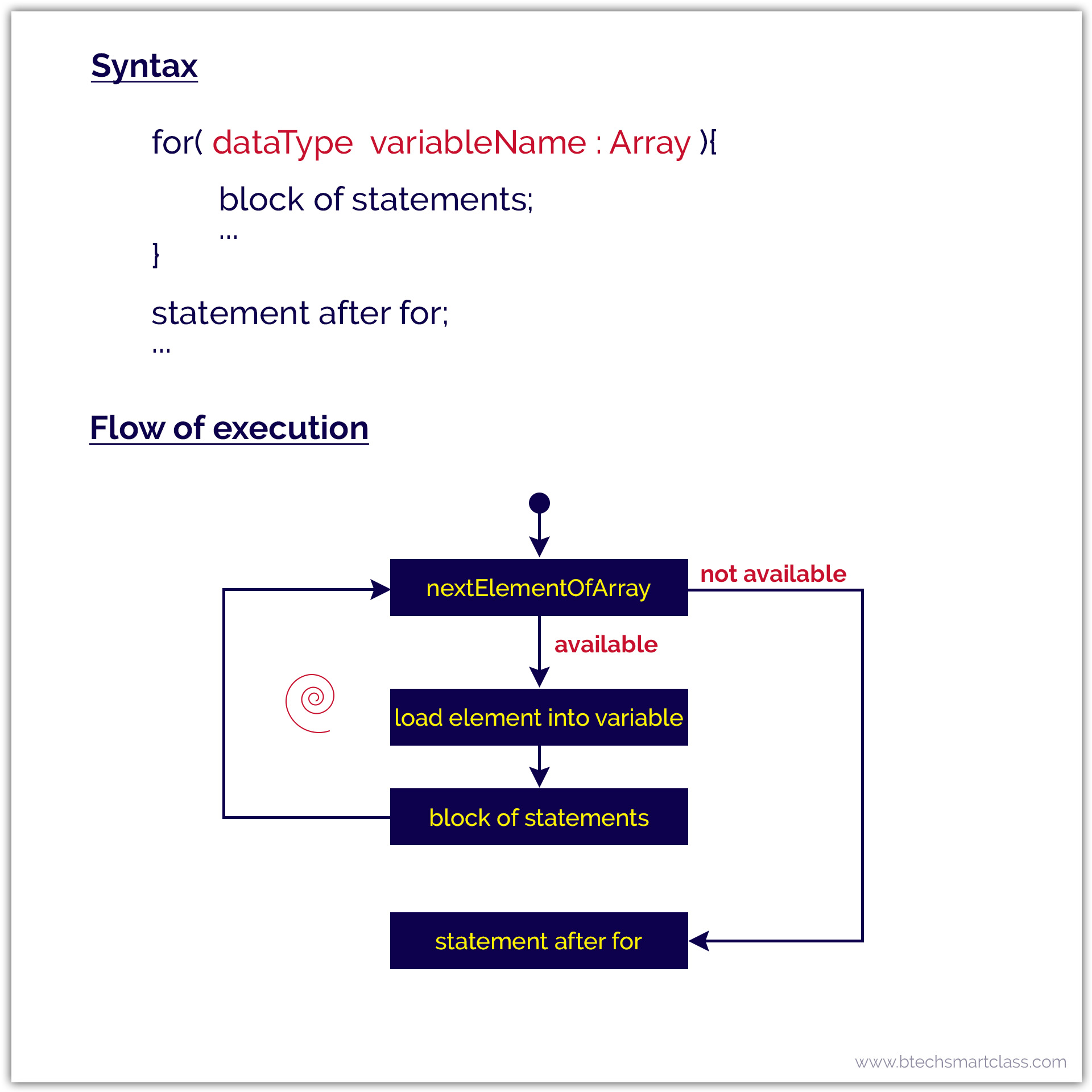Let's look at the following example java code.

Java Program
``````public class ForEachTest {

public static void main(String[] args) {

int[] arrayList = {10, 20, 30, 40, 50};

for(int i : arrayList) {
System.out.println("i = " + i);
}

System.out.println("Statement after for-each!");
}

}```
```

When we run this code, it produce the following output.